# 6.7 Integer exponents and scientific notation  (Page 4/10)

 Page 4 / 10

Simplify: $\frac{{x}^{8}}{{x}^{-3}}.$

${x}^{11}$

Simplify: $\frac{{y}^{8}}{{y}^{-6}}.$

${y}^{13}$

## Convert from decimal notation to scientific notation

Remember working with place value for whole numbers and decimals? Our number system is based on powers of 10. We use tens, hundreds, thousands, and so on. Our decimal numbers are also based on powers of tens—tenths, hundredths, thousandths, and so on. Consider the numbers 4,000 and $0.004$ . We know that 4,000 means $4\phantom{\rule{0.2em}{0ex}}×\phantom{\rule{0.2em}{0ex}}1,000$ and 0.004 means $4\phantom{\rule{0.2em}{0ex}}×\phantom{\rule{0.2em}{0ex}}\frac{1}{1,000}$ .

If we write the 1000 as a power of ten in exponential form, we can rewrite these numbers in this way:

$\begin{array}{cccc}4,000\hfill & & & \phantom{\rule{4em}{0ex}}0.004\hfill \\ 4\phantom{\rule{0.2em}{0ex}}×\phantom{\rule{0.2em}{0ex}}1,000\hfill & & & \phantom{\rule{4em}{0ex}}4\phantom{\rule{0.2em}{0ex}}×\phantom{\rule{0.2em}{0ex}}\frac{1}{1,000}\hfill \\ 4\phantom{\rule{0.2em}{0ex}}×\phantom{\rule{0.2em}{0ex}}{10}^{3}\hfill & & & \phantom{\rule{4em}{0ex}}4\phantom{\rule{0.2em}{0ex}}×\phantom{\rule{0.2em}{0ex}}\frac{1}{{10}^{3}}\hfill \\ & & & \phantom{\rule{4em}{0ex}}4\phantom{\rule{0.2em}{0ex}}×\phantom{\rule{0.2em}{0ex}}{10}^{-3}\hfill \end{array}$

When a number is written as a product of two numbers, where the first factor is a number greater than or equal to one but less than 10, and the second factor is a power of 10 written in exponential form, it is said to be in scientific notation.

## Scientific notation

A number is expressed in scientific notation    when it is of the form

It is customary in scientific notation to use as the $\phantom{\rule{0.2em}{0ex}}×\phantom{\rule{0.2em}{0ex}}$ multiplication sign, even though we avoid using this sign elsewhere in algebra.

If we look at what happened to the decimal point, we can see a method to easily convert from decimal notation to scientific notation    .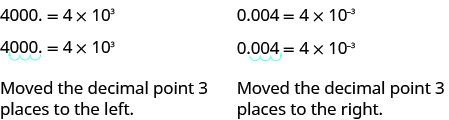In both cases, the decimal was moved 3 places to get the first factor between 1 and 10.

$\begin{array}{cccc}\text{The power of 10 is positive when the number is larger than 1:}\hfill & & & 4,000=4\phantom{\rule{0.2em}{0ex}}×\phantom{\rule{0.2em}{0ex}}{10}^{3}\hfill \\ \text{The power of 10 is negative when the number is between 0 and 1:}\hfill & & & 0.004=4\phantom{\rule{0.2em}{0ex}}×\phantom{\rule{0.2em}{0ex}}{10}^{-3}\hfill \end{array}$

## How to convert from decimal notation to scientific notation

Write in scientific notation: 37,000.

## Solution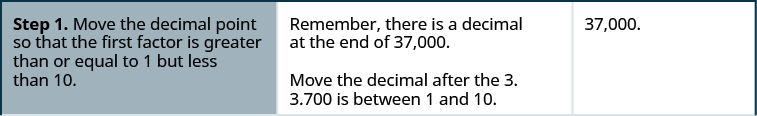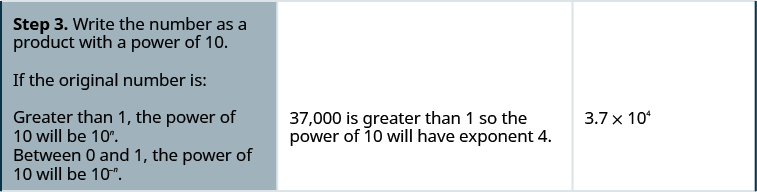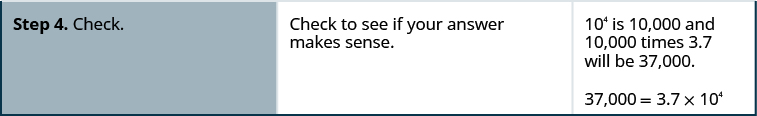Write in scientific notation: $96,000.$

$9.6\phantom{\rule{0.2em}{0ex}}×\phantom{\rule{0.2em}{0ex}}{10}^{4}$

Write in scientific notation: $48,300.$

$4.83\phantom{\rule{0.2em}{0ex}}×\phantom{\rule{0.2em}{0ex}}{10}^{4}$

## Convert from decimal notation to scientific notation

1. Move the decimal point so that the first factor is greater than or equal to 1 but less than 10.
2. Count the number of decimal places, n , that the decimal point was moved.
3. Write the number as a product with a power of 10.
If the original number is:
• greater than 1, the power of 10 will be 10 n .
• between 0 and 1, the power of 10 will be 10 −n .
4. Check.

Write in scientific notation: $0.0052.$

## Solution

The original number, $0.0052$ , is between 0 and 1 so we will have a negative power of 10.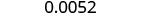Move the decimal point to get 5.2, a number between 1 and 10.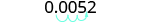Count the number of decimal places the point was moved.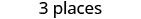Write as a product with a power of 10.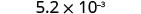Check. $\begin{array}{}\\ \\ \phantom{\rule{3em}{0ex}}5.2\phantom{\rule{0.2em}{0ex}}×\phantom{\rule{0.2em}{0ex}}{10}^{-3}\hfill \\ \phantom{\rule{3em}{0ex}}5.2\phantom{\rule{0.2em}{0ex}}×\phantom{\rule{0.2em}{0ex}}\frac{1}{{10}^{3}}\hfill \\ \phantom{\rule{3em}{0ex}}5.2\phantom{\rule{0.2em}{0ex}}×\phantom{\rule{0.2em}{0ex}}\frac{1}{1000}\hfill \\ \phantom{\rule{3em}{0ex}}5.2\phantom{\rule{0.2em}{0ex}}×\phantom{\rule{0.2em}{0ex}}0.001\hfill \end{array}$ $\phantom{\rule{2em}{0ex}}0.0052$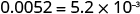Write in scientific notation: $0.0078.$

$7.8\phantom{\rule{0.2em}{0ex}}×\phantom{\rule{0.2em}{0ex}}{10}^{-3}$

Write in scientific notation: $0.0129.$

$1.29\phantom{\rule{0.2em}{0ex}}×\phantom{\rule{0.2em}{0ex}}{10}^{-2}$

## Convert scientific notation to decimal form

How can we convert from scientific notation to decimal form? Let’s look at two numbers written in scientific notation and see.

$\begin{array}{cccc}\hfill 9.12\phantom{\rule{0.2em}{0ex}}×\phantom{\rule{0.2em}{0ex}}{10}^{4}\hfill & & & \hfill \phantom{\rule{4em}{0ex}}9.12\phantom{\rule{0.2em}{0ex}}×\phantom{\rule{0.2em}{0ex}}{10}^{-4}\hfill \\ \hfill 9.12\phantom{\rule{0.2em}{0ex}}×\phantom{\rule{0.2em}{0ex}}10,000\hfill & & & \hfill \phantom{\rule{4em}{0ex}}9.12\phantom{\rule{0.2em}{0ex}}×\phantom{\rule{0.2em}{0ex}}0.0001\hfill \\ \hfill 91,200\hfill & & & \hfill \phantom{\rule{4em}{0ex}}0.000912\hfill \end{array}$

If we look at the location of the decimal point, we can see an easy method to convert a number from scientific notation to decimal form.

$\begin{array}{cccc}\hfill 9.12\phantom{\rule{0.2em}{0ex}}×\phantom{\rule{0.2em}{0ex}}{10}^{4}=91,200\hfill & & & \hfill \phantom{\rule{4em}{0ex}}9.12\phantom{\rule{0.2em}{0ex}}×\phantom{\rule{0.2em}{0ex}}{10}^{-4}=0.000912\hfill \end{array}$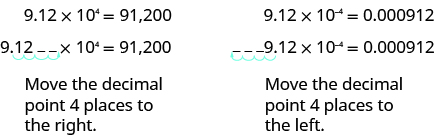In both cases the decimal point moved 4 places. When the exponent was positive, the decimal moved to the right. When the exponent was negative, the decimal point moved to the left.

John left his house in Irvine at 8:35 am to drive to a meeting in Los Angeles, 45 miles away. He arrived at the meeting at 9:50. At 3:30 pm, he left the meeting and drove home. He arrived home at 5:18.
p-2/3=5/6 how do I solve it with explanation pls
P=3/2
Vanarith
1/2p2-2/3p=5p/6
James
Cindy
is y=7/5 a solution of 5y+3=10y-4
yes
James
Cindy
Lucinda has a pocketful of dimes and quarters with a value of $6.20. The number of dimes is 18 more than 3 times the number of quarters. How many dimes and how many quarters does Lucinda have? Rhonda Reply Find an equation for the line that passes through the point P ( 0 , − 4 ) and has a slope 8/9 . Gabriel Reply is that a negative 4 or positive 4? Felix y = mx + b Felix if negative -4, then -4=8/9(0) + b Felix -4=b Felix if positive 4, then 4=b Felix then plug in y=8/9x - 4 or y=8/9x+4 Felix Macario is making 12 pounds of nut mixture with macadamia nuts and almonds. macadamia nuts cost$9 per pound and almonds cost $5.25 per pound. how many pounds of macadamia nuts and how many pounds of almonds should macario use for the mixture to cost$6.50 per pound to make?
Nga and Lauren bought a chest at a flea market for $50. They re-finished it and then added a 350 % mark - up Makaila Reply$1750
Cindy
the sum of two Numbers is 19 and their difference is 15
2, 17
Jose
interesting
saw
4,2
Cindy
Felecia left her home to visit her daughter, driving 45mph. Her husband waited for the dog sitter to arrive and left home 20 minutes, or 13 hour later. He drove 55mph to catch up to Felecia. How long before he reaches her?
integer greater than 2 and less than 12
2 < x < 12
Felix
I'm guessing you are doing inequalities...
Felix
Actually, translating words into algebraic expressions / equations...
Felix
hi
Darianna
hello
Mister
Eric here
Eric
6
Cindy
He charges $125 per job. His monthly expenses are$1,600. How many jobs must he work in order to make a profit of at least \$2,400?
at least 20
Ayla
what are the steps?
Alicia
6.4 jobs
Grahame
32
Grahame
1600+2400= total amount with expenses. 4000/125= number of jobs needed to make that min profit of 2400. answer is 32
Orlando
He must work 32 jobs to make a profit
POP
32
Cindy
what is algebra
repeated addition and subtraction of the order of operations. i love algebra I'm obsessed.
Shemiah
hi
Krekar
Eric here. I'm a parent. 53 years old. I have never taken algebra. I want to learn.
Eric
I am 63 and never learned algebra
Cindy
One-fourth of the candies in a bag of M&M’s are red. If there are 23 red candies, how many candies are in the bag?
they are 92 candies in the bag
POP
92
Cindy
rectangular field solutions
What is this?
Donna
t
muqtaar
the proudact of 3x^3-5×^2+3 and 2x^2+5x-4 in z7[x]/ is
?
Choli
a rock is thrown directly upward with an initial velocity of 96feet per second from a cliff 190 feet above a beach. The hight of tha rock above the beach after t second is given by the equation h=_16t^2+96t+190
Usman

#### Get Jobilize Job Search Mobile App in your pocket Now!By Anonymous UserBy OpenStaxByBy OpenStaxBy Madison ChristianBy Joli JuliannaBy Ryan LoweBy JavaChamp TeamBy Cath YuBy Brooke Delaney